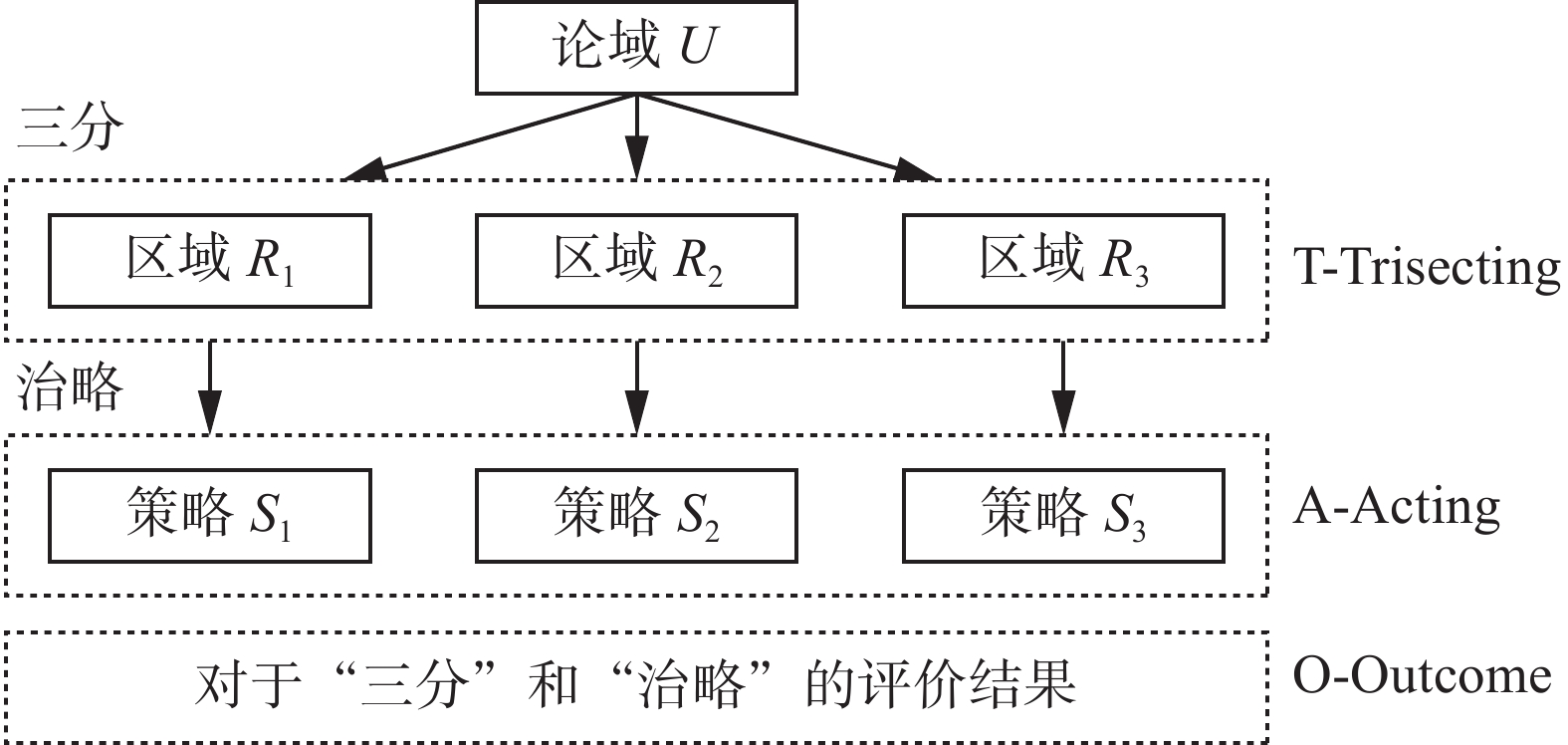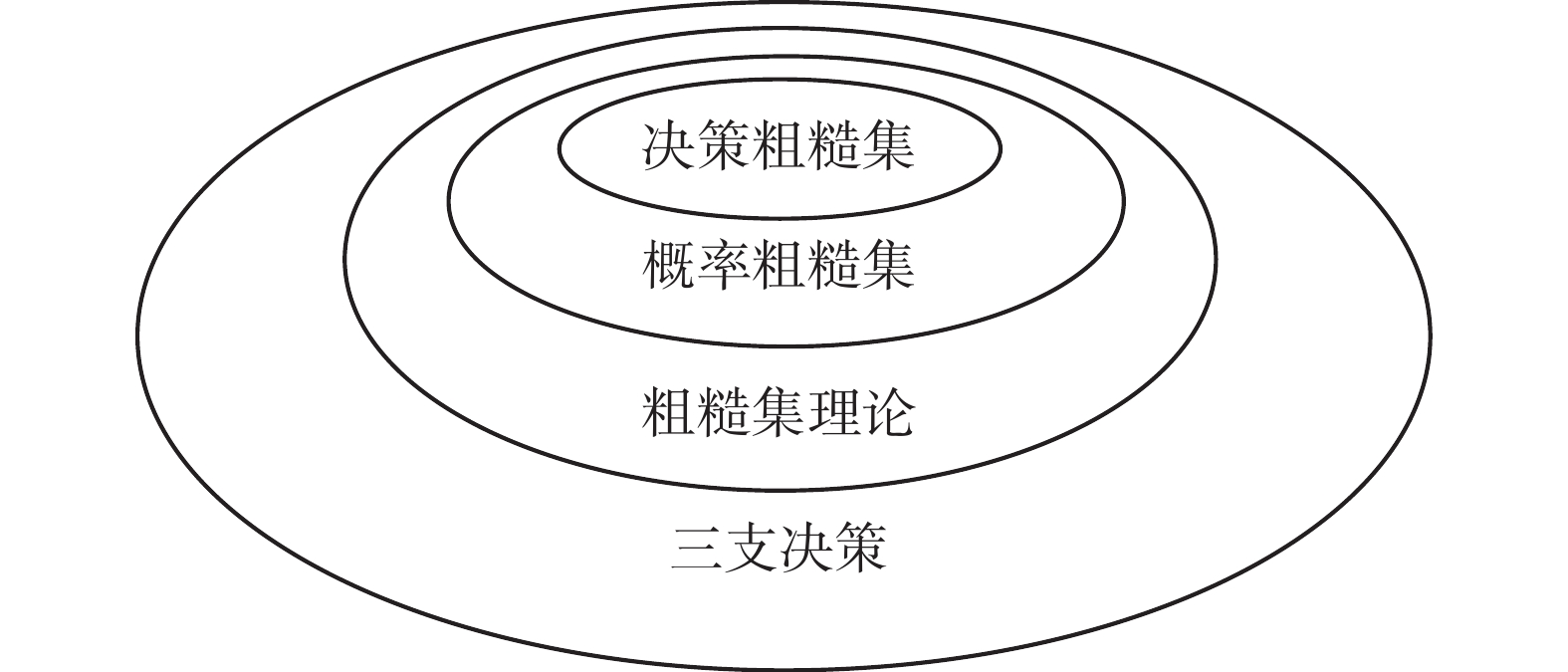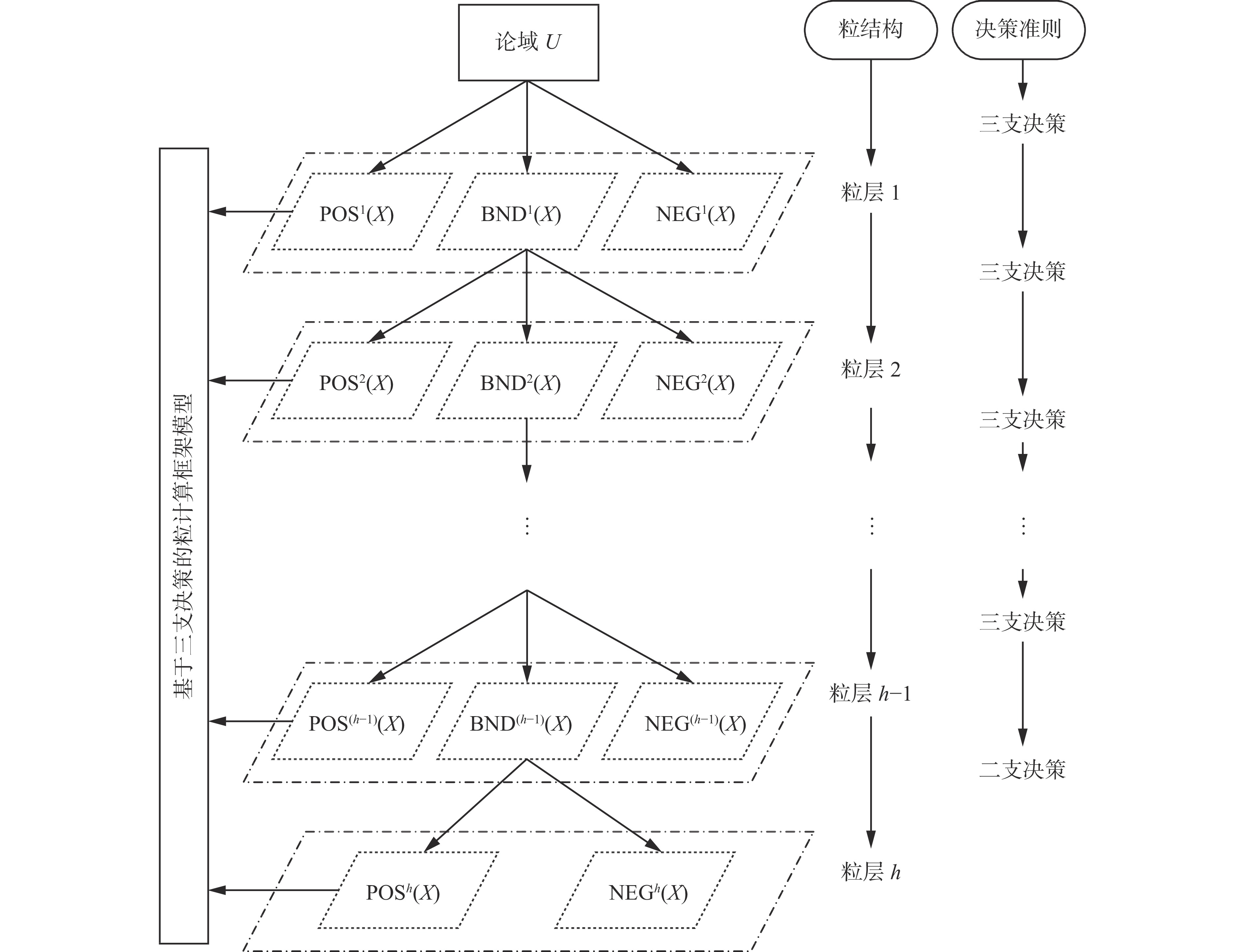﻿ 三支决策−基于粗糙集与粒计算研究视角
«上一篇文章快速检索 高级检索

 智能系统学报2019, Vol. 14Issue (6): 1111-1120  DOI: 10.11992/tis.2019050390

### 引用本文LIU Dun, LI Tianrui, YANG Xin, et al. Three-way decisions: research perspectives for rough sets and granular computing[J]. CAAI Transactions on Intelligent Systems, 2019, 14(6): 1111-1120. DOI: 10.11992/tis.201905039.### 文章历史

1. 西南交通大学 经济管理学院，四川 成都 610031;
2. 西南交通大学 信息科学与技术学院，四川 成都 611756;
3. 西南财经大学 经济信息工程学院，四川 成都 611130;
4. 电子科技大学 经济与管理学院，四川 成都 610054

Three-way decisions: research perspectives for rough sets and granular computing
LIU Dun 1, LI Tianrui 2, YANG Xin 3, LIANG Decui 4
1. School of Economics and Management, Southwest Jiaotong University, Chengdu 610031, China;
2. School of Information Science and Technology, Southwest Jiaotong University, Chengdu 611756, China;
3. School of Economic Information Engineering, Southwest University of Finance and Economics, Chengdu 611130, China;
4. School of Management and Economics, University of Electronic Science and Technology of China, Chengdu 610054, China
Abstract: With the developments of three historical stages on three-way decisions (3WD), this paper introduces the development track and evolution process of 3WD. Firstly, the historical context, internal connections and relations between 3WD and rough sets are carefully investigated. The inclusion relation among decision-theoretic rough sets, probabilistic rough sets, rough sets and 3WD is also discussed. Secondly, we discuss the methodology of 3WD via granular computing with two strategies of " multi-level” and " multi-view”. Thirdly, we propose a novel framework model of granular computing with 3WD. Finally, this paper gives the research status and future research directions of 3WD.
Key words: three-way decisions    granular computing    rough set theory    multi-level    multi-view    multi-granularity    probabilistic rough sets    decision-theoretic rough sets    learning cost

1 三支决策基本模型与框架

 $f\;:\;U \to \{ {R_1},{R_2},{R_3}\}$ (1)

 $\begin{array}{l} R_1^c = {R_2} \cup {R_3}\\ R_{\rm{2}}^c = {R_{\rm{1}}} \cup {R_3}\\ R_{\rm{3}}^c = {R_{\rm{1}}} \cup {R_{\rm{2}}} \end{array}$ (2)Download: 图 1 三支决策TAO模型框架图 Fig. 1 The TAO model of three-way decisions

 $\begin{gathered} {R_1}(v(x)) = \{ x \in U|\,\,v(x)\,\geqslant \,\alpha \} \\ {R_2}(v(x)) = \{ x \in U|\beta < v(x) < \alpha \} \\ {R_3}(v(x)) = \{ x \in U|v(x)\,\,\leqslant \,\,\beta \} \end{gathered}$ (3)

 $\bar v(x) = {w_1}{v_{{c_1}}}(x) + {w_2}{v_{{c_2}}}(x) + \cdots + {w_n}{v_{{c_m}}}(x)$ (4)

 $\begin{gathered} {R_1}(\bar v(x)) = \{ x \in U|\,\,\bar v(x)\, \geqslant M\} \\ {R_2}(\bar v(x)) = \{ x \in U|N < \bar v(x) < M\} \\ {R_3}(\bar v(x)) = \{ x \in U|\bar v(x)\,\, \leqslant N\} \end{gathered}$ (5)

 $\Re (\alpha ,\beta ) = a{\Re _1}(\alpha ,\beta ) + b{\Re _2}(\alpha ,\beta ) + c{\Re _3}(\alpha ,\beta )$ (6)

 $\arg \mathop {\min }\limits_{(\alpha ,\beta )} \Re (\alpha ,\beta )$ (7)

2 三支决策、粗糙集与决策粗糙集

 $\left\{\begin{gathered} \underline {\rm apr} (X) = \left\{ {x \in U|[x] \subseteq X} \right\}; \\ \overline {\rm apr} (X) = \left\{ {x \in U|[x] \cap X \ne \text{Ø} } \right\} = \\ \left\{ {x \in U|\neg ([x] \subseteq {X^c})} \right\} \\ \end{gathered} \right.$ (8)

 $\left\{\begin{gathered} \rm{POS}(X) = \underline {\rm apr} (X) = \{ x \in U|[x] \subseteq X\} ; \\ { \rm BND}(X) = \overline {\rm apr} (X) - \underline {\rm apr} (X) \\ \{ x \in U|\neg ([x] \subseteq X\} \wedge \neg ([x] \subseteq {X^c})\} ; =\\ {\rm NEG}(X) = U - \overline {\rm apr} (X) = \\ \{ x \in U|[x] \subseteq {X^c}\} \\ \end{gathered} \right.$ (9)

 $\begin{array}{l} {\underline {\rm apr} _{(\alpha ,\;\beta )}}(X) = \{ x \in U|{\rm Pr}(X|[x]) \geqslant \alpha \}\\ {\overline {\rm apr} _{(\alpha ,\;\beta )}}(X) = \{ x \in U|{\rm Pr}(X|[x]) > \beta \} \end{array}$ (10)

 $\begin{gathered} {\rm POS}{_{(\alpha ,\;\beta )}}(X) = \{ x \in U|{\rm Pr}(X|[x]) \geqslant \alpha \}\\ {\rm BND}{_{(\alpha ,\;\beta )}}(X) = \{ x \in U|\beta < {\rm Pr}(X|[x]) < \alpha \}\\ {\rm NEG}{_{(\alpha ,\;\beta )}}(X) = \{ x \in U|{\rm Pr}(X|[x]) \leqslant \beta \} \end{gathered}$ (11)

 $\begin{gathered} R({a_P}|[x]) = {{\text{λ}} _{PP}}{\rm{Pr}}(X|[x]) + {{\text{λ}} _{PN}}{\rm{Pr}}(\neg X|[x]) \\ R({a_B}|[x]) = {{\text{λ}} _{BP}}{\rm{Pr}}(X|[x]) + {{\text{λ}} _{BN}}{\rm{Pr}}(\neg X|[x]) \\ R({a_N}|[x]) = {{\text{λ}} _{NP}}{\rm{Pr}}(X|[x]) + {{\text{λ}} _{NN}}{\rm{Pr}}(\neg X|[x]) \end{gathered}$ (12)

(P) $R({a_P}|[x]) \leqslant R({a_B}|[x])$ $R({a_P}|[x]) \leqslant R({a_N}|[x])$ 同时成立，则 $x \in {\rm POS}(X)$

(B) $R({a_B}|[x]) \leqslant R({a_P}|[x])$ $R({a_B}|[x]) \leqslant R({a_N}|[x])$ 同时成立，则 $x \in {\rm BND}(X)$

(N) $R({a_N}|[x]) \leqslant R({a_P}|[x])$ $R({a_N}|[x]) \leqslant R({a_B}|[x])$ 同时成立，则 $x \in {\rm NEG}(X)$

(P1)：如果 ${\rm{Pr}}(X|[x]) \geqslant \alpha$ ，则： $x \in {\rm{POS}}(X)$

(B1)：如果 $\beta < {\rm{Pr}}(X|[x]) < \alpha$ ，则： $x \in {\rm{BND}}(X)$

(N1)：如果 ${\rm{Pr}}(X|[x]) \leqslant \beta$ ，则： $x \in {\rm{NEG}}(X)$

 $\begin{gathered} \alpha \, = \,\dfrac{{({{\textit{λ}} _{PN}} - {{\textit{λ}} _{BN}})}}{{({{\textit{λ}} _{PN}} - {{\textit{λ}} _{BN}}) + ({{\textit{λ}} _{BP}} - {{\textit{λ}} _{PP}})}}\,\\ \beta \, = \,\dfrac{{({{\textit{λ}} _{BN}} - {{\textit{λ}} _{NN}})}}{{({{\textit{λ}} _{BN}} - {{\textit{λ}} _{NN}}) + ({{\textit{λ}} _{NP}} - {{\textit{λ}} _{PP}})}}\,\\ \gamma = \,\dfrac{{({{\textit{λ}} _{PN}} - {{\textit{λ}} _{NN}})}}{{({{\textit{λ}} _{PN}} - {{\textit{λ}} _{NN}}) + ({{\textit{λ}} _{NP}} - {{\textit{λ}} _{PP}})}}\, \end{gathered}$ (13)Download: 图 2 三支决策与相关粗糙集模型间的关系 Fig. 2 The relations between three-way decisions and rough set models
3 三支决策的粒计算方法Download: 图 3 基于多层次视角下的粒度变化过程 Fig. 3 The granularity variation process based on multilevel perspective

 $({\rm{5}} \times \sqrt {\rm{5}} \times \sqrt {\rm{5}} )\,{\rm{ - }}\,(\sqrt {\rm{5}} \div \sqrt {\rm{5}} \,)\,=\,\,{\rm{24}}$

4 三支决策的粒计算框架模型Download: 图 4 基于三支决策的粒计算框架模型 Fig. 4 The framework model of granular computing with three-way decisions

 $\begin{gathered} {\rm{BND}}{^k}(X)\,=\,\,{\rm{POS}}{^{k{\rm{ + 1}}}}(X) \cup {\rm{BND}}{^{k{\rm{ + 1}}}}(X) \cup {\rm{NEG}}{^{k{\rm{ + 1}}}}(X)\\ {\rm{POS}}{^k}(X) \subseteq {\rm{POS}}{^{k{\rm{ + 1}}}}(X)\\ |{\rm{POS}}{^k}(X)|\,\, \leqslant \,\,|{\rm{POS}}{^{k{\rm{ + 1}}}}(X)|\\ {\rm{NEG}}{^k}(X) \subseteq {\rm{NEG}}{^{k{\rm{ + 1}}}}(X)\\ |{\rm{NEG}}{^k}(X)|\; \leqslant \;|{\rm{NEG}}{^{k{\rm{ + 1}}}}(X)|\\ {\rm{BND}}{^{k{\rm{ + 1}}}}(X) \subseteq {\rm{BND}}{^k}(X)\\ |{\rm{BND}}{^{k{\rm{ + 1}}}}(X)|\; \leqslant \;|{\rm{BND}}{^k}(X)| \end{gathered}$ (13)

5 结束语

  YAO Yiyu. Three-way decisions with probabilistic rough sets[J]. Information sciences, 2010, 180(3): 341-353. DOI:10.1016/j.ins.2009.09.021 (0)  YAO Yiyu. Three-way decision and granular computing[J]. International journal of approximate reasoning, 2018, 103: 107-123. DOI:10.1016/j.ijar.2018.09.005 (0)  YAO Y Y, WONG S K M. A decision theoretic framework for approximating concepts[J]. International journal of man-machine studies, 1992, 37(6): 793-809. DOI:10.1016/0020-7373(92)90069-W (0)  刘盾, 李天瑞, 李华雄. 粗糙集理论: 基于三支决策视角[J]. 南京大学学报(自然科学版), 2013, 49(5): 574-581. LIU Dun, LI Tianrui, LI Huaxiong. Rough set theory: a three-way decisions perspective[J]. Journal of Nanjing University (Natural Sciences), 2013, 49(5): 574-581. (0)  刘盾, 梁德翠. 广义三支决策与狭义三支决策[J]. 计算机科学与探索, 2017, 11(3): 502-510. LIU Dun, LIANG Decui. Generalized three-way decisions and special three-way decisions[J]. Journal of frontiers of computer science & technology, 2017, 11(3): 502-510. (0)  刘盾, 李天瑞, 梁德翠, 等. 三支决策的时空性[J]. 智能系统学报, 2019, 14(1): 141-149. LIU Dun, LI Tianrui, LIANG Decui, et al. The temporality and spatiality of three-way decisions[J]. CAAI transactions on intelligent systems, 2019, 14(1): 141-149. (0)  HU Baoqing. Three-way decisions space and three-way decisions[J]. Information sciences, 2014, 281: 21-52. DOI:10.1016/j.ins.2014.05.015 (0)  LI Xiaonan, YI Huangjian, SHE Yanhong, et al. Generalized three-way decision models based on subset evaluation[J]. International journal of approximate reasoning, 2017, 83: 142-159. DOI:10.1016/j.ijar.2017.01.005 (0)  YAO Yiyu. Three-way decisions and cognitive computing[J]. Cognitive computation, 2016, 8(4): 543-554. DOI:10.1007/s12559-016-9397-5 (0)  LI Jinhai, HUANG Chenchen, QI Jianjun, et al. Three-way cognitive concept learning via multi-granularity[J]. Information sciences, 2017, 378: 244-263. DOI:10.1016/j.ins.2016.04.051 (0)  DAVIDE C, DIDIER D. A map of dependencies among three-valued logics[J]. Information sciences, 2013, 250: 162-177. DOI:10.1016/j.ins.2013.06.040 (0)  QI Jianjun, QIAN Ting, WEI Ling. The connections between three-way and classical concept lattices[J]. Knowledge-based systems, 2016, 91: 143-151. DOI:10.1016/j.knosys.2015.08.006 (0)  LIANG Decui, LIU Dun. Systematic studies on three-way decisions with interval-valued decision-theoretic rough sets[J]. Information sciences, 2014, 276: 186-203. DOI:10.1016/j.ins.2014.02.054 (0)  LIANG Decui, LIU Dun, PEDRYCZ W, et al. Triangular fuzzy decision-theoretic rough sets[J]. International journal of approximate reasoning, 2013, 54(8): 1087-1106. DOI:10.1016/j.ijar.2013.03.014 (0)  LIANG Decui, LIU Dun. Deriving three-way decisions from intuitionistic fuzzy decision-theoretic rough sets[J]. Information sciences, 2015, 300: 28-48. DOI:10.1016/j.ins.2014.12.036 (0)  LIU Dun, LI Tianrui, LIANG Decui. Three-way decisions in stochastic decision-theoretic rough sets[M]// PETERSJ F, SKOWRON A, LI Tianrui, et al. Transactions on Rough Sets XVIII. Berlin, Heidelberg: Springer, 2014: 110−130. (0)  LI Huaxiong, ZHOU Xianzhong, ZHAO Jiabao, et al. Non-monotonic attribute reduction in decision-theoretic rough sets[J]. Fundamenta informaticae, 2013, 126(4): 415-432. DOI:10.3233/FI-2013-889 (0)  JIA Xiuyi, LIAO Wenhe, TANG Zhenmin, et al. Minimum cost attribute reduction in decision-theoretic rough set models[J]. Information sciences, 2013, 219: 151-167. DOI:10.1016/j.ins.2012.07.010 (0)  MIN Fan, HE Huaping, QIAN Yuhua, et al. Test-cost-sensitive attribute reduction[J]. Information sciences, 2011, 181(22): 4928-4942. DOI:10.1016/j.ins.2011.07.010 (0)  ZHANG Xianyong, MIAO Duoqian. Three-layer granular structures and three-way informational measures of a decision table[J]. Information sciences, 2017, 412−413: 67-86. DOI:10.1016/j.ins.2017.05.032 (0)  REN Ruisi, WEI Ling. The attribute reductions of three-way concept lattices[J]. Knowledge-based systems, 2016, 99: 92-102. DOI:10.1016/j.knosys.2016.01.045 (0)  YU Hong, LIU Zhanguo, WANG Guoyin. An automatic method to determine the number of clusters using decision-theoretic rough set[J]. International journal of approximate reasoning, 2014, 55(1): 101-115. DOI:10.1016/j.ijar.2013.03.018 (0)  ZHOU Bing. Multi-class decision-theoretic rough sets[J]. International journal of approximate reasoning, 2014, 55(1): 211-224. DOI:10.1016/j.ijar.2013.04.006 (0)  LIU Dun, LI Tianrui, LIANG Decui. Incorporating logistic regression to decision-theoretic rough sets for classifications[J]. International journal of approximate reasoning, 2014, 55(1): 197-210. DOI:10.1016/j.ijar.2013.02.013 (0)  LI Yuefeng, ZHANG Libiao, XU Yue, et al. Enhancing binary classification by modeling uncertain boundary in three-way decisions[J]. IEEE transactions on knowledge and data engineering, 2017, 29(7): 1438-1451. DOI:10.1109/TKDE.2017.2681671 (0)  ZHANG Libo, LI Huaxiong, ZHOU Xianzhong, et al. Sequential three-way decision based on multi-granular autoencoder features[J]. Information sciences, 2020, 507: 630-643. DOI:10.1016/j.ins.2019.03.061 (0)  ZHANG Hengru, MIN Fan, SHI Bing. Regression-based three-way recommendation[J]. Information sciences, 2017, 378: 444-461. DOI:10.1016/j.ins.2016.03.019 (0)  WANG Min, MIN Fan, ZHANG Zhiheng, et al. Active learning through density clustering[J]. Expert systems with applications, 2017, 85: 305-317. DOI:10.1016/j.eswa.2017.05.046 (0)  ZHOU Bing, YAO Yiyu, LUO Jigang. Cost-sensitive three-way email spam filtering[J]. Journal of intelligent information systems, 2014, 42(1): 19-45. DOI:10.1007/s10844-013-0254-7 (0)  LI Huaxiong, ZHANG Libo, HUANG Bing, et al. Sequential three-way decision and granulation for cost-sensitive face recognition[J]. Knowledge-based systems, 2016, 91: 241-251. DOI:10.1016/j.knosys.2015.07.040 (0)  LI Y, ZHANG C, SWAN J R. An information filtering model on the web and its application in JobAgent[J]. Knowledge-based systems, 2000, 13(5): 285-296. DOI:10.1016/S0950-7051(00)00088-5 (0)  WOODWARD P W, NAYLOR J C. An application to Bayesian methods in SPC[J]. The statistician, 1993, 42(4): 461-469. DOI:10.2307/2348478 (0)  LIU Dun, LI Tianrui, LIANG Decui. Three-way government decision analysis with Decision-theoretic rough sets[J]. International journal of uncertainty, fuzziness and knowledge-based systems, 2012, 20(S1): 119-132. (0)  贾修一, 商琳, 周献中, 等. 三支决策理论与应用[M]. 南京: 南京大学出版社, 2012. JIA Xiuyi, SHANG Lin, ZHOU Xianzhong, et al. The method and theory of three-way decisions[M]. Nanjing: Nanjing University Press, 2012. (0)  刘盾, 李天瑞, 苗夺谦, 等. 三支决策与粒计算[J]. 北京: 科学出版社, 2013. LIU Dun, LI Tianrui, MIAO Duoqian, et al. Three-way decisions and granular computing[M]. Beijing: Science Press, 2013. (0)  于洪, 王国胤, 李天瑞, 等. 三支决策: 复杂问题求解方法与实践[M]. 北京: 科学出版社, 2015. YU Hong, WANG Guoyin, LI Tianrui, et al. Three-way decisions: methods and practices for complex problem solving[M]. Beijing: Science Press, 2015. (0)  张燕平, 姚一豫, 苗夺谦, 等. 粒计算、商空间及三支决策的回顾与发展[M]. 北京: 科学出版社, 2017. ZHANG Yanping, YAO Yiyu, MIAO Duoqian, et al. Review and development of granular computing, quotient space and three decisions[M]. Beijing: Science Press, 2017. (0)  叶晓庆, 刘盾, 梁德翠. 基于协同过滤的三支粒推荐算法研究[J]. 计算机科学, 2018, 45(1): 90-96. YE Xiaoqing, LIU Dun, LIANG Decui. Three-way granular recommendation algorithm based on collaborative filtering[J]. Computer science, 2018, 45(1): 90-96. (0)